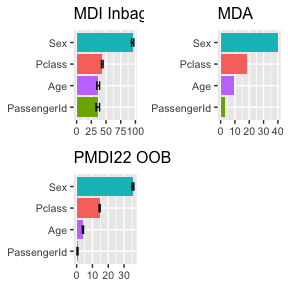# Variable Importance based on reduction of Gini on OOB

## Variable importance in trees

Variable importance is not very well defined as a concept. Even for the case of a linear model with $$n$$ observations, $$p$$ variables and the standard $$n >> p$$ situation, there is no theoretically defined variable importance metric in the sense of a parametric quantity that a variable importance estimator should try to estimate. Variable importance measures for random forests have been receiving increased attention in bioinformatics, for instance to select a subset of genetic markers relevant for the prediction of a certain disease. They also have been used as screening tools in important applications highlighting the need for reliable and well-understood feature importance measures.

The default choice in most software implementations of random forests is the mean decrease in impurity (MDI). The MDI of a feature is computed as a (weighted) mean of the individual trees’ improvement in the splitting criterion produced by each variable. A substantial shortcoming of this default measure is its evaluation on the in-bag samples which can lead to severe overfitting . It was also pointed out by Strobl et al. that the variable importance measures of Breiman’s original Random Forest method … are not reliable in situations where potential predictor variables vary in their scale of measurement or their number of categories. There have been multiple attempts at correcting the well understood bias of the Gini impurity measure both as a split cirterion as well as a contributor to importance scores, each one coming from a different perspective. Strobl et al. derive the exact distribution of the maximally selected Gini gain along with their resulting p-values by means of a combinatorial approach. Shi et al. suggest a solution to the bias for the case of regression trees as well as binary classification trees which is also based on P-values. Several authors argue that the criterion for split variable and split point selection should be separated.

We use the well known titanic data set to illustrate the perils of putting too much faith into the Gini importance which is based entirely on training data - not on OOB samples - and makes no attempt to discount impurity decreases in deep trees that are pretty much frivolous and will not survive in a validation set. In the following model we include passengerID as a feature along with the more reasonable Age, Sex and Pclass.

#### Penalized Gini Impurity applied to Titanic data

The Figure below show both measures of variable importance and (maybe?) surprisingly passengerID turns out to be ranked number $$3$$ for the Gini importance (MDI). This troubling result is robust to random shuffling of the ID.

naRows = is.na(titanic_train$Age) data2=titanic_train[!naRows,] RF =randomForest(Survived ~ Age + Sex + Pclass + PassengerId, data=data2, ntree=50,importance=TRUE,mtry=2, keep.inbag=TRUE) if (is.factor(data2$Survived)) data2$Survived = as.numeric(data2$Survived)-1
VI_PMDI3 = GiniImportanceForest(RF, data2,score="PMDI22",Predictor=mean)
plotVI2(VI_PMDI3, score="PMDI22", decreasing = TRUE)